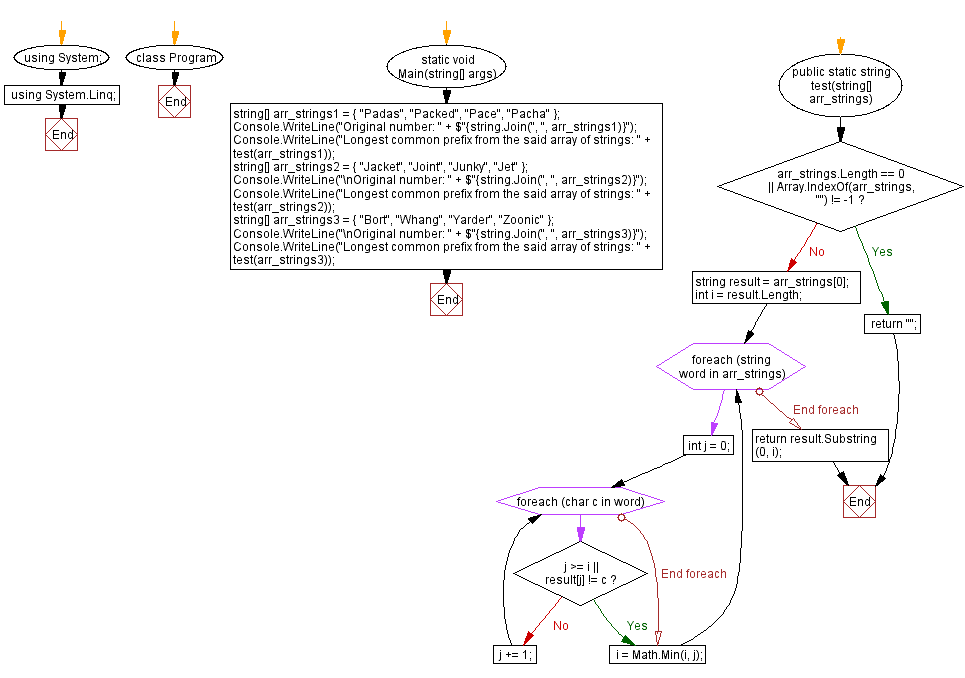﻿ C# - Find the longest common prefix from an array of strings# C# Sharp Exercises: Find the longest common prefix from an array of strings

## C# Sharp Basic: Exercise-94 with Solution

Write a C# program that finds the longest common prefix from an array of strings.

Sample Data:
({ "Padas", "Packed", "Pace", "Pacha" }) -> "Pa"
({ "Jacket", "Joint", "Junky", "Jet" }) -> "J"
({ "Bort", "Whang", "Yarder", "Zoonic" }) -> ""

Sample Solution:

C# Sharp Code:

`````` using System;
using System.Linq;
namespace exercises
{
class Program
{
static void Main(string[] args)
{
string[] arr_strings1 = { "Padas", "Packed", "Pace", "Pacha" };
Console.WriteLine("Original number: " + \$"{string.Join(", ", arr_strings1)}");
Console.WriteLine("Longest common prefix from the said array of strings: " + test(arr_strings1));
string[] arr_strings2 = { "Jacket", "Joint", "Junky", "Jet" };
Console.WriteLine("\nOriginal number: " + \$"{string.Join(", ", arr_strings2)}");
Console.WriteLine("Longest common prefix from the said array of strings: " + test(arr_strings2));
string[] arr_strings3 = { "Bort", "Whang", "Yarder", "Zoonic" };
Console.WriteLine("\nOriginal number: " + \$"{string.Join(", ", arr_strings3)}");
Console.WriteLine("Longest common prefix from the said array of strings: " + test(arr_strings3));
}
public static string test(string[] arr_strings)
{
if (arr_strings.Length == 0 || Array.IndexOf(arr_strings, "") != -1)
return "";
string result = arr_strings;
int i = result.Length;
foreach (string word in arr_strings)
{
int j = 0;
foreach (char c in word)
{
if (j >= i || result[j] != c)
break;
j += 1;
}
i = Math.Min(i, j);
}
return result.Substring(0, i);
}
}
}
``````

Sample Output:

```Original number: Padas, Packed, Pace, Pacha
Longest common prefix from the said array of strings: Pa

Original number: Jacket, Joint, Junky, Jet
Longest common prefix from the said array of strings: J

Original number: Bort, Whang, Yarder, Zoonic
Longest common prefix from the said array of strings:
```

Flowchart:C# Sharp Code Editor:

Improve this sample solution and post your code through Disqus

Previous C# Sharp Exercise: Square root of a given number.
Next C# Sharp Exercise: Verify that a string contains valid parentheses.

What is the difficulty level of this exercise?

﻿